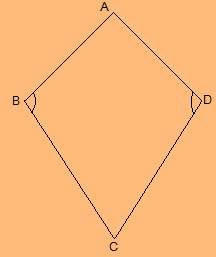# Draw a rough figure of a quadrilateral that is not a parallelogram but has exactly two opposite angles of equal measure.ABCD is a figure of quadrilateral which is not a parallelogram but has exactly two opposite angles

that is ∠B = ∠D of equal measure.

It is not a parallelogram as ∠A ≠ ∠C.